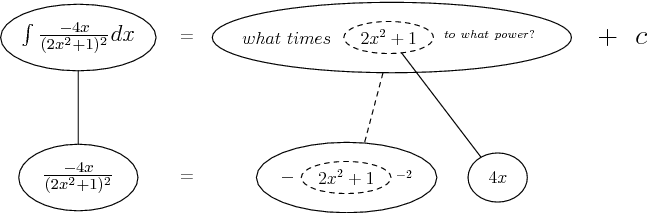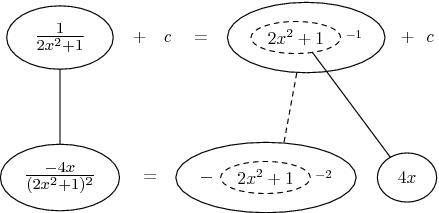# Thread: [SOLVED] integration

1. ## [SOLVED] integration

$\displaystyle \int \frac{-4x}{4x^4+4x^2+1}$

$\displaystyle \int \frac{-4x}{(2x^2+1)(2x^2+1)}$

What would my next steps be to complete this integral?

2. Use the substitution $\displaystyle 2x^2+1=t\Rightarrow 4xdx=dt$

3. $\displaystyle \int\frac{-4x}{t^2}*\frac{dt}{4x}$

$\displaystyle \int\frac{1}{(2x^2+1)(2x^2+1)}$

is this correct?

4. And, if a picture helps...Straight continuous lines differentiate/integrate with respect to x, the straight dashed line with respect to the dashed balloon expression - as though it were a variable like u.

Don't integrate - balloontegrate! Balloon Calculus: worked examples from past papers

5. As my answer I got $\displaystyle y=\frac{1}{2x^2+1}+C$

6. Yep

7. thanks

8.Originally Posted by ronaldo_07As my answer I got $\displaystyle y=\frac{1}{2x^2+1}+C$
You can always differentiate it and see if you get back the integrand.

9. Yes - and of course the differentiation is the same picture (completed) but read downwards instead of up.Don't integrate - balloontegrate! http://www.ballooncalculus.org/examples.png

#### Search Tags

integration, solved